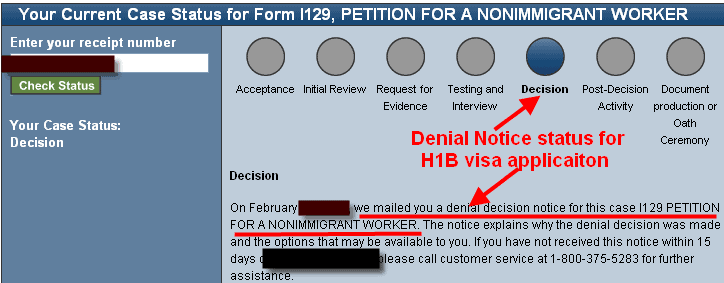# Mean, Mode & MedianSince H1 Visas & immigration seems to be the hot topic making buzz now, Lets learn how do they arrive at median median for US workers and H1B workers , Will be using Python for the purpose

We need numpy

if you have python installed on your computer all you need is

sudo apt get pip

pip install numpy

What is Mean, Median and Mode

Mean

Lets say we have 5 H1B workers earning 60000, 70000, 90000,12000,150000

We represent these data in what we call lists in python

H1B=[60000, 70000, 90000,12000,150000]

Mean = Sum of salaries of all H1B employees / # of employees

Mean = (60000 + 70000 + 90000 +12000 + 150000) /  5

Python code using numpy

# H1 visa mean income
import numpy as np
salary=[60000, 70000, 90000,12000,150000]
np.mean(salary)

76400.0

Median

Lets say we have 5 H1B workers earning 60000, 70000, 90000,12000,150000

Median = sort the values and take the value at midpoint

Median = sort [60000, 70000, 90000,12000,150000] and select midpoint

# H1 visa mean income
import numpy as np
salary=[60000, 70000, 90000,12000,150000]
np.median(salary)

70000

Mode

most common value in dataset

H1B=[60000, 70000, 90000,12000,150000,60000]

Each value

60000:2

70000:1

90000:1

12000:1

150000:1

Mode = 60000

Lets generate some random H1B employee salaries with minimum base salary of \$60000 and maximum of \$200000 ( As per 2015 data only 5% of H1B make tier 4 wage which is above 150000)

# H1 visa lottery pick for ppl with 60K-200K base salaries
import numpy as np
h1b_salary = np.random.randint(60000,high=200000,size=20000)
print h1b_salary
Untitled

`[128495 125554 141888 ...,  92709  66694 152183]`
Lets compute mode
from scipy import stats
stats.mode(h1b_salary)
`ModeResult(mode=array(), count=array())`
what next